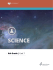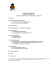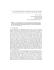# Calculating Work

## Transcription

Calculating Work
```TEKS 7.7A contrast situations where work is done with different amounts of force to situations where no work is done such as
moving a box with a ramp and without a ramp, or standing still
Calculating Work
The equation to solve work problems looks like this:
Work (W) = force (F) x distance (d) or
W=Fxd
The SI (metric) unit for force is Newton (N).
The SI (metric) unit for distance is meter (m).
The SI (metric) unit for work is the newton-meter which is
called a joule (J).
Sample Problem: A fork lift is used to
lift a box and move it across a
warehouse. The 1,023N box is lifted
4m. How much work is done by the fork
lift?
• Step 1:
Write down the equation needed to solve the
problem.
W=Fxd
• Step 2: Insert all known measurements into the
equation.
W = (1,023N) x (4m)
• Step 3: Solve carefully.
W = 4,092 J
The forklift does 4,092 J of work.
• Step 4: Make sure that all of your numbers have the
correct SI unit label.
(Remember as you solve the following problems to keep
following the steps shown on the previous page.)
Problem #1: How much work is done by a person who
uses a force of 27N to move a grocery cart 12m?
Show work here:
Show work here:
Problem #2: If an upward force
of 2,200N is used to lift a box 2
meters, how much work was
Show work here:
Problem #3: You and a friend apply
a combined force of 500N to push a
piano 3 meters. How much work was
done to move the piano?
Get ready for some work practice problems! Make sure to
label all numbers with SI units and show all of your work.
#1. Calculate the amount of work done to
lift a crate weighing 567N a distance of
20m.
#2. A strong wind exerts a force of 25 N
on a trash can blowing it 3 m. How much
work is done on the trash can?
#3. A bulldozer uses a force of 2000 N to
push a mound of dirt 3 m. How much
work did the bulldozer do?
#4. A student exerts 170 N of force to
push a desk chair 4m across a floor, how
much work would be done?
#5. A rock climber with a weight of 150 N
climbs 8 m up a rock wall. How much
work did the climber do?
#6. A girl uses a force of 12N to pull her
sled 4m up a hill. How much work did it
take to pull the sled?
Bonus: A young boy applied a force of 2,550N on his St. Bernard dog who is sitting on
the boy's tennis shoes. He was unable to move the dog. Explain how much work he did
while trying to push the dog.
```

### front suspension lift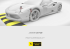### FRONT SUSPENSION LIFT### Product Brochure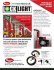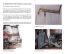### Below Packer Application: Dead String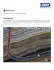### SlideAwayLift Wheelchair Lift Brochure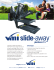### Specification Guide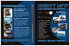### suspension lift kit new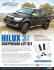### LIFT-2K® LIFT-25K - Cisco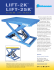### Car-Lifts - NANI – Verladetechnik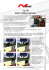### DH-ESU 5000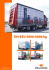### San Mateo County - The Campaign for Grade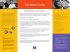### 1 | Page Southeast Area Transit District Maintenance Scissors Lift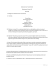### science 607